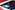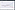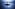# Макс(*аргументы, ключ = ...). Как изменить ключ, чтобы получить только определенные значения?

Я немного запутался с функцией `max(*args, key = ..)`. Представьте, что у меня есть следующий список: `my_list = [2, 3, 4, -5, -2, -1]`. Я хочу найти максимальное значение среди отрицательных значений в my_list. Для этого я использую следующий код `max(my_list, key = lambda x: x<0)`, но он дает мне `-5`. Не могли бы вы объяснить мне логику?

Сначала отфильтруйте список только до отрицательных значений, а затем найдите в нем максимум. В настоящее время значения просто сравниваются по «истине/ложи», что делает результат более или менее случайным.

22.04.2022 18:54

`key` определяет, какой как сравнивать каждый элемент, а не если для их сравнения. `key=lambda x: x<0` эквивалентно «представить, что значение равно `True`, если отрицательное, `False`, если положительное». Обратите внимание, что `False < True` верно, а `False < False` ложно.

22.04.2022 18:56
`key` is to enable custom comparisons. The code that compares might be as follows `if key(current_value) > key(max_value)`.
22.04.2022 18:57

Can you please elaborate a bit. I am just trying to understand the logic how max(..., key =) works in my case

22.04.2022 18:57
`key` turns each value into another value (in your case `True` or `False`), and the max of those values determines which value from the list is returned.
22.04.2022 18:59Анализ настроения постов в Twitter с помощью Python, Tweepy и Flair7 лайфхаков для начинающих Python-программистовУстановка Apache Cassandra на Mac OSСертификатная программа "Кванты Python": Бэктестер ансамблевых методов на основе ООПСоздание персонального файлового хранилищаСоздание приборной панели для анализа данных на GCP - часть I
1
5
42
4
Данный вопрос помечен как решенный

## Ответы 4

What you are doing when calling `max(my_list, key = lambda x: x<0)` is saying: "which value in the list is largest, but compare the value of the result for the expression (x<0) for each element instead of the element's value." Therefore, the `max` operator basically sees the list as `[False, False, False, True, True, True]` when deciding which is biggest and in python this is equivalent to `[0, 0, 0, 1, 1, 1]`. `max` returns the first maximum element it sees, which corresponds to -5.

You likely want to filter the list to only negative values first, then call `max` on the result

I would use a list comprehension to filter out the positive values and then find the maximum.

``````max([i for i in my_list if i < 0])
``````

The `[]` are superfluous, without them a generator expression would be more efficient.

22.04.2022 19:01

While I wouldn't use `max` alone for this, you could, with an appropriate `key` function that doesn't collapse multiple values:

``````max(my_list, key=lambda x: -float('inf') if x > 0 else x)
``````

This causes all positive values to compare as if they were negative infinity, which cannot be the largest value in a list that contains any non-positive values.

(If all the values are all positive, you'll need to adapt this in some way, because it will still return the first value in the list: no successive value is bigger. Unless requested, I consider that beyond the scope of the question as currently stated.)

Ответ принят как подходящий

Yes, you CAN do this by a single line with `max` function. I explain it below.

And ALSO there is a better way than list comprehension that I explained in the end.

This is how the max function works if you have provided the key parameter. It will loop through the values of the list and then it applies the lambda function (named `key`) on each value. So all of the values will be mapped to the result of the lambda function. Then it performs the `max` function on these results.

So when you have `key=lambda x: x<0` this will return `True` or `False` as `x<0` is a boolean expression. So the result list would be like `[False, False, False, True, True, True]`. These `False` and `True` values are processed as `0` and `1` values for the max function.

The `max` function gets `[0, 0, 0, 1, 1, 1]` and it finds the first one that is the maximum number in the list (number 1) that is the first `1` in the list that corresponds to number -5 in the original list.

### Solution:

There are multiple ways to find the maximum negative number like using a for loop or list comprehension or even combining `filter` and `max`. But, to understand the `max` function and it's parameters better I show you the way you can do the thing you want (find the maximum negative number) by the `max` function.

``````max(my_list, key = lambda x: min(my_list) if x>=0 else x)
``````

This code will find the maximum negative number.

##### How does this code work?

The provided lambda expression will map all the number as this:

If the number is positive we replace it's value for the max function as the minimum number so it will never be chosen as the maximum negative number. (We could do this by removing all the negative number by `filter` but I decided to do all the job just with lambda to show you the way.)

If the number is negative it will keep it's own value. So for your example that the list is `my_list = [2, 3, 4, -5, -2, -1]` it will create `[-5, -5, -5, -5, -2, -1]` and then it will perform the `max` function on this list that it will find the `-1` number that is what you want.

I wish it could help you understand how to find the maximum negative number just by the `max` function and the way maximum function works.

NOTE: This code is not optimal. Because every time it computes the minimum value. This code is only for learning purposes.

### Best way to do this

I believe a better code is this:

``````max((num for num in my_list if num<0))
``````

Note that this is not list comprehension. It is better than list comprehension. Instead it creates a generator that only process one value at a time. This is better that list comprehension because list comprehension creates the whole list at once and then send it to the max function. But this code process each value at a time and don't fill the memory with a big list. So this is more efficient.

This repeatedly runs through the list to find the minimum. Not really efficient.

22.04.2022 19:30

@deceze Yes I know. Because it find the minimum every time. But I wrote this code just for learning purposes. The better way is list comprehension as I always use.

22.04.2022 19:40

@deceze I updated the answer. Mentioned that why the first way it is not ideal AND also mentioned a better way to do this. Please see the last part of the answer.

22.04.2022 20:23